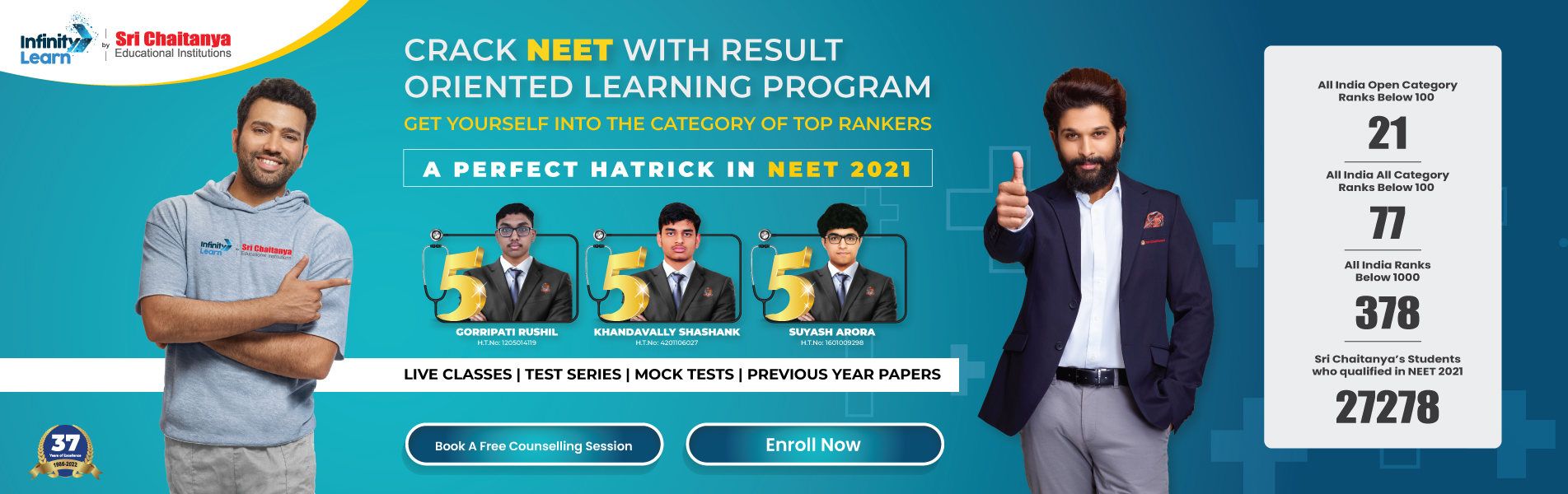Practical Geometry Class 6 Notes Maths Chapter 14

# Practical Geometry Class 6 Notes Maths Chapter 14Infinity Learn NEET IIT-JEE
Infinity Learn NEET
banner-chaitanya-web

We use the following tools in drawing geometrical shapes:

• The compasses
• The divider
• Set-squares, and
• The protractor.

In this chapter, we shall consider only “Ruler-and-compass constructions”.

Free IIT-JEE/NEET/NCERT/CBSE Material

Download 100,000+ FREE PDFs, solved questions, Previous Year Papers, Quizzes, and Puzzles directly to your Whatsapp account!

Circle
Every point on the boundary of a circle is at an equal distance from its center.

Construction of a circle when the length of its radius is known
We need to use compasses here.

A Line Segment
We need a ruler here.

Construction of a line segment of a given length
We have to use a ruler for this construction.

Constructing a copy of a given line segment
We may use a ruler or trace paper here. But it is better than we would use ruler and compasses for making, the construction.

Perpendiculars
Two lines or rays or line segments are said to be perpendicular if they intersect such that the angle formed between them is 90°.

Perpendicular to a line through a point on it
It may be drawn in three ways:

• by paper folding,
• using a ruler and a set-square, and
• using a ruler and the compasses.

Perpendicular to a line through a point not on it
Either of the three methods given below may be adopted:

• by paper folding,
• using a ruler and a set-square, and
• using a ruler and the compasses.

The perpendicular bisector of a line segment
There are three methods as given below:

• by paper folding,
• by using transparent tapes, and
• by using ruler and compasses.

Angles
Constructing an angle of a given measure
We use a ruler and a protractor here.

Constructing a copy of an angle of unknown measure
We use a ruler (or a straight edge) and the compasses.

Bisector of an angle
Two methods are possible:

• by paper folding
• by using ruler and compasses

Angles of special measures
There are some elegant and accurate methods to construct some angles of special sizes without using the protractor.
Some of such constructions are:

• Constructing a 60° angle
• Constructing a 30° angle
• Constructing a 120° angle
• Constructing a 150° angle
• Constructing a 90° angle
• Constructing a 45° angle

Join Infinity Learn Regular Class Programme!